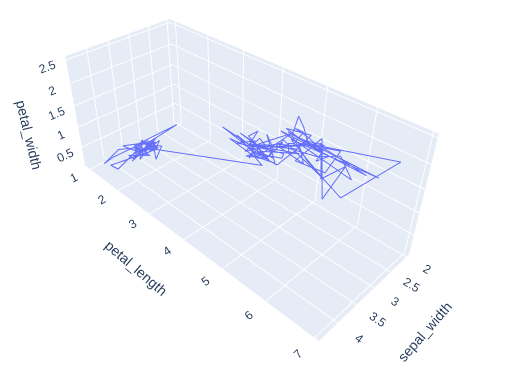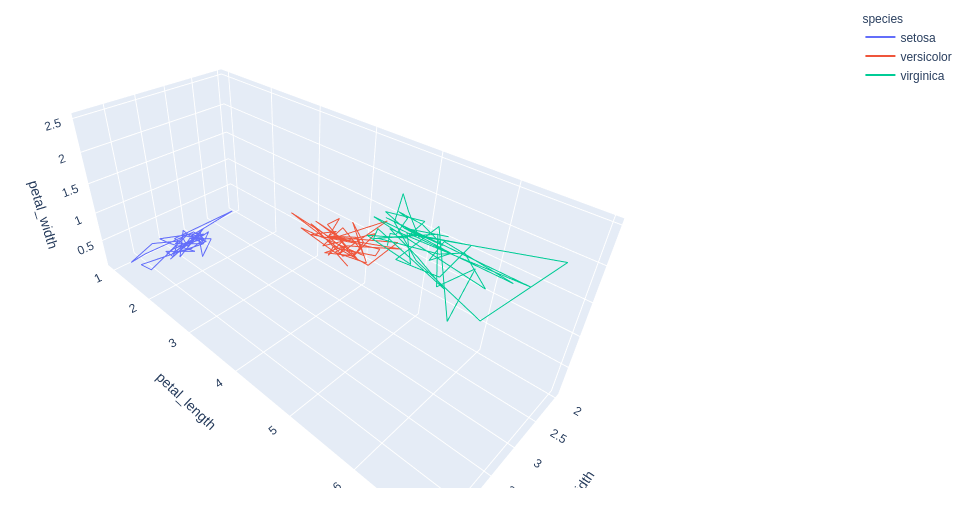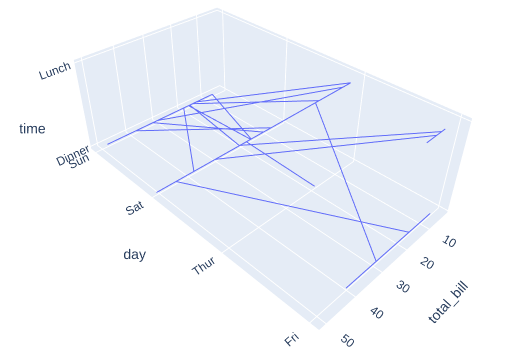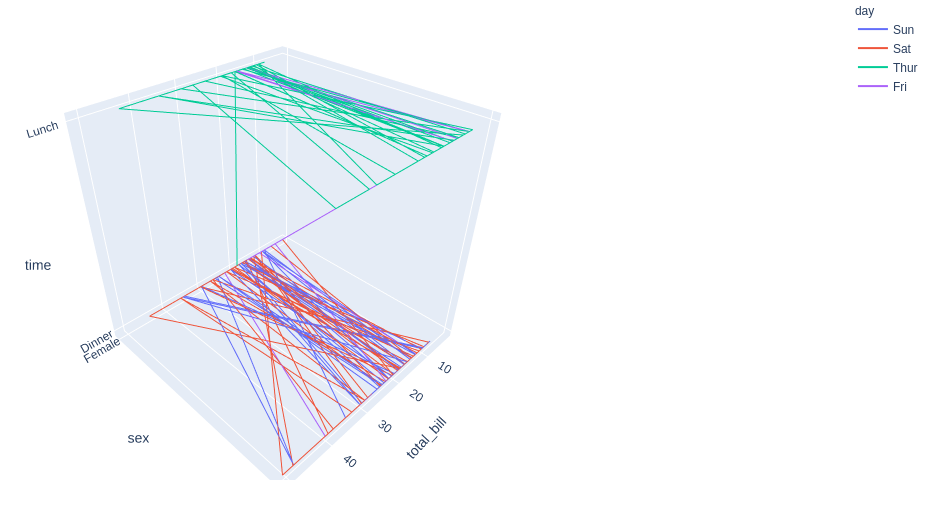# 3D Line Plots using Plotly in Python

Plotly is a Python library that is used to design graphs, especially interactive graphs. It can plot various graphs and charts like histogram, barplot, boxplot, spreadplot and many more. It is mainly used in data analysis as well as financial analysis. plotly is an interactive visualization library

## Line Plot in Plotly

Line plot in plotly is much accessible and illustrious annexation to plotly which manage a variety of types of data and assemble easy-to-style statistic. With px.line_3d each data position is represented as a vertex  (which location is given by the x, y and z columns) of a polyline mark in 3D space. Line chart Displays a series of numerical data as points which are connected by lines. It visualizes to show two data trends. The main productive feature is it can display thousands of data points without scrolling. It can be created using the line_3d() method of plotly.express class.

Syntax: plotly.express.line_3d(data_frame=None, x=None, y=None, z=None, color=None, line_dash=None, text=None, line_group=None, hover_name=None, hover_data=None, custom_data=None, error_x=None, error_x_minus=None, error_y=None, error_y_minus=None, error_z=None, error_z_minus=None, animation_frame=None, animation_group=None, category_orders={}, labels={}, color_discrete_sequence=None, color_discrete_map={}, line_dash_sequence=None, line_dash_map={}, log_x=False, log_y=False, log_z=False, range_x=None, range_y=None, range_z=None, title=None, template=None, width=None, height=None

Parameters:

data_frame: This argument needs to be passed for column names (and not keyword names) to be used.

x, y, z: Either a name of a column in data_frame, or a pandas Series or array_like object. Values from this column or array_like are used to position marks along the x, y and z axis respectively in cartesian coordinates.

color: Either a name of a column in data_frame, or a pandas Series or array_like object. Values from this column or array_like are used to assign color to marks.

Example 1: Using Iris dataset

## Python3

 `import` `plotly.express as px ` ` `  ` `  `df ``=` `px.data.iris() ` ` `  `fig ``=` `px.line_3d(df, x``=``"sepal_width"``, ` `                 ``y``=``"petal_length"``,  ` `                 ``z``=``"petal_width"``) ` `fig.show()`

Output:Example 2: Iris dataset with color argument

## Python3

 `import` `plotly.express as px ` ` `  ` `  `df ``=` `px.data.iris() ` ` `  `fig ``=` `px.line_3d(df, x``=``"sepal_width"``, ` `                 ``y``=``"petal_length"``,  ` `                 ``z``=``"petal_width"``,  ` `                 ``color``=``"species"``) ` `fig.show()`

Output:Example 3: Using tips dataset

## Python3

 `import` `plotly.express as px ` ` `  ` `  `df ``=` `px.data.tips() ` ` `  `fig ``=` `px.line_3d(df, x``=``"total_bill"``,  ` `                 ``y``=``"day"``, z``=``"time"``) ` `fig.show()`

Output:Example 4: Tips dataset with color argument.

## Python3

 `import` `plotly.express as px ` ` `  ` `  `df ``=` `px.data.tips() ` ` `  `fig ``=` `px.line_3d(df, x``=``"total_bill"``, y``=``"day"``, z``=``"time"``) ` `fig.show()`

Output:Whether you're preparing for your first job interview or aiming to upskill in this ever-evolving tech landscape, GeeksforGeeks Courses are your key to success. We provide top-quality content at affordable prices, all geared towards accelerating your growth in a time-bound manner. Join the millions we've already empowered, and we're here to do the same for you. Don't miss out - check it out now!

Previous
Next